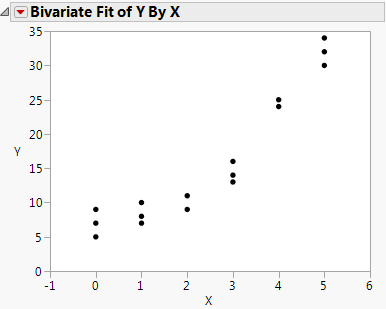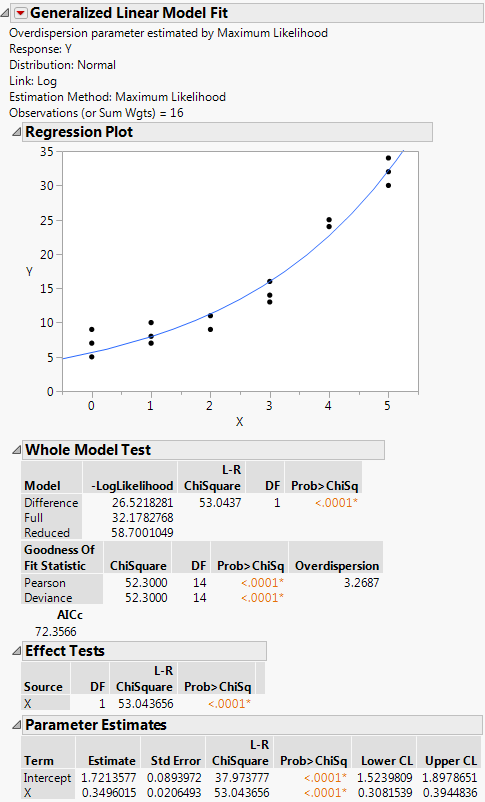Publication date: 03/23/2021

## Normal Regression with a Log Link

This example uses the Nor.jmp data table, where X is an explanatory variable and Y is the response variable. First, you explore the relationship between X and Y to determine the appropriate link function to use in the Generalized Linear Model personality of the Fit Model platform.

1. Select Help > Sample Data Library and open Nor.jmp.

2. Select Analyze > Fit Y By X.

3. Select Y and click Y, Response.

4. Select X, click X, Factor, and then click OK.

Figure 12.8 Y by X Results for Nor.jmpYou can see that Y varies nonlinearly with X and that the variance is approximately constant. Therefore, a normal distribution with a log link function is appropriate to model these data; that is, log(μi) = xi'β so that μi = exp(xi'β).

5. Select Analyze > Fit Model.

6. In the Personality list, select the Generalized Linear Model.

7. In the Distribution list, select Normal.

8. In the Link Function list, select Log.

9. Select Y and click Y.

10. Select X and click Add.

11. Click Run.

Figure 12.9 Nor ResultsWant more information? Have questions? Get answers in the JMP User Community (community.jmp.com).